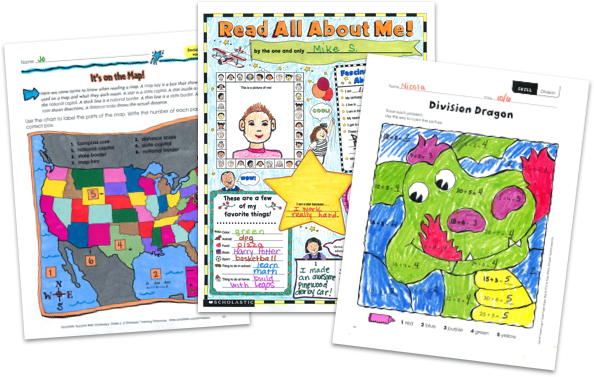MY FILE CABINETSaved to File Cabinet
×

# Math Worksheets from Scholastic TeachablesScholastic Teachables (formerly Scholastic Printables) has over 5,700 math worksheets for grades Pre-K through 8 that span more than 25 different math skills! Sign up today and get instant online access to math worksheets, lessons, practice pages, and more to cover all grades and skills.

Scholastic Teachables (formerly Scholastic Printables) has over 5,700 math worksheets for grades Pre-K through 8 that span more than 25 different math skills! Sign up today and get instant online access to math worksheets, lessons, practice pages, and more to cover all grades and skills.

## Scholastic's Math Worksheet Covers:

• Charts and Graphs
• Data Analysis
• Decimals
• Early Math
• Estimation
• Fractions
• Geometry
• Glyphs
• Logic and Problem Solving
• Math Fluency and Intervention
• Money
• Multiplication and Division
• Numbers and Counting
• Number Sense
• Order of Operations
• Patterns
• Percents, Ratios, Proportions and Scale
• Place Value
• Probability & Statistics
• Real-World Math
• Time and Measurement
• Types of Numbers
• Word Problems
• And More!

## Sample Math Worksheets

More than 5,700 printable math worksheets from Scholastic cover 25-plus math topics from Pre-K through Grade 8. Sign up and you'll also get access to Scholastic's more than 30,000 award-winning printable resources in math, reading, science, and social studies.

Here's a sample of worksheets to try in your class—FREE with a 30-day trial or subscription.

## Scholastic's Math Worksheet Covers:

• Charts and Graphs
• Data Analysis
• Decimals
• Early Math
• Estimation
• Fractions
• Geometry
• Glyphs
• Logic and Problem Solving
• Math Fluency and Intervention
• Money
• Multiplication and Division
• Numbers and Counting
• Number Sense
• Order of Operations
• Patterns
• Percents, Ratios, Proportions and Scale
• Place Value
• Probability & Statistics
• Real-World Math
• Time and Measurement
• Types of Numbers
• Word Problems
• And More!
• Charts and Graphs
• Data Analysis
• Decimals
• Early Math
• Estimation
• Fractions
• Geometry
• Glyphs
• Logic and Problem Solving
• Math Fluency and Intervention
• Money
• Multiplication and Division
• Numbers and Counting
• Number Sense
• Order of Operations
• Patterns
• Percents, Ratios, Proportions and Scale
• Place Value
• Probability & Statistics
• Real-World Math
• Time and Measurement
• Types of Numbers
• Word Problems
• And More!

## Sample Math Worksheets

More than 5,700 printable math worksheets from Scholastic cover 25-plus math topics from Pre-K through Grade 8. Sign up and you'll also get access to Scholastic's more than 30,000 award-winning printable resources in math, reading, science, and social studies.

Here's a sample of worksheets to try in your class—FREE with a 30-day trial or subscription.## Additional Alphabet Worksheets (Subscription Required)

Try FREE for 30 days!

If you are not 100% satisfied cancel during the FREE trial and owe nothing. If you are not 100% satisfied cancel during the FREE trial and owe nothing.